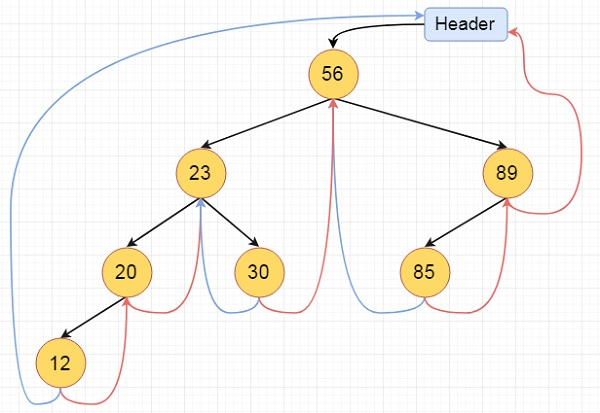# Inorder Traversal of a Threaded Binary Tree in C++

Here we will see the threaded binary tree data structure. We know that the binary tree nodes may have at most two children. But if they have only one children, or no children, the link part in the linked list representation remains null. Using threaded binary tree representation, we can reuse that empty links by making some threads.

If one node has some vacant left or right child area, that will be used as thread. There are two types of threaded binary tree. The single threaded tree or fully threaded binary tree.

For fully threaded binary tree, each node has five fields. Three fields like normal binary tree node, another two fields to store Boolean value to denote whether link of that side is actual link or thread.

This is the fully threaded binary tree## Algorithm

inorder():
Begin
temp := root
repeat infinitely, do
p := temp
temp = right of temp
if right flag of p is false, then
while left flag of temp is not null, do
temp := left of temp
done
end if
if temp and root are same, then
break
end if
print key of temp
done
End

## Example

Live Demo

#include <iostream>
#define MAX_VALUE 65536
using namespace std;
class N { //node declaration
public:
int k;
N *l, *r;
bool leftTh, rightTh;
};
private:
N *root;
public:
ThreadedBinaryTree() { //constructor to initialize the variables
root= new N();
root->r= root->l= root;
root->leftTh = true;
root->k = MAX_VALUE;
}
void insert(int key) {
N *p = root;
for (;;) {
if (p->k< key) { //move to right thread
if (p->rightTh)
break;
p = p->r;
}
else if (p->k > key) { // move to left thread
if (p->leftTh)
break;
p = p->l;
}
else {
return;
}
}
N *temp = new N();
temp->k = key;
temp->rightTh= temp->leftTh= true;
if (p->k < key) {
temp->r = p->r;
temp->l= p;
p->r = temp;
p->rightTh= false;
}
else {
temp->r = p;
temp->l = p->l;
p->l = temp;
p->leftTh = false;
}
}
void inorder() { //print the tree
N *temp = root, *p;
for (;;) {
p = temp;
temp = temp->r;
if (!p->rightTh) {
while (!temp->leftTh) {
temp = temp->l;
}
}
if (temp == root)
break;
cout<<temp->k<<" ";
}
cout<<endl;
}
};
int main() {
tbt.insert(56);
tbt.insert(23);
tbt.insert(89);
tbt.insert(85);
tbt.insert(20);
tbt.insert(30);
tbt.insert(12);
tbt.inorder();
cout<<"\n";
}

## Output

Threaded Binary Tree
12 20 23 30 56 85 89